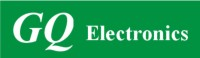Active Users: / Visits Today:
Highest Active Users:
GQ Electronics Technical Support Forum
Home | Profile | Register | Active Topics | Members | Search | FAQAll Forums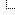GQ Electronics Forums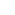3. GQ EMF EF Meter RF Spectrum Power Analyzer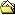Density Gain and CSV Export

Note: You must be registered in order to post a reply.

Screensize:
Format Mode: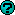Format: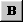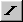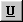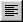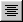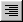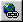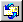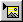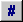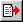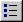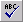Message:

* HTML is OFF
* Forum Code is ON
 Smilies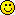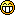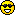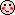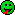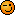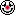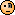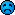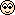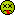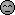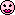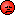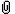Insert an Image File
Check here to include your profile signature.

 T O P I C    R E V I E W frank.mathieu@GQ Posted - 08/07/2021 : 13:39:40 Hello, I do not intend to change the standard setting. However, I'd like to understand the meaning of 10 decibel Density Gain correctly.Pls. let me ask two questions:1st Question:Assuming that (without Density Gain) 0db equal 1mW/m˛, do 0db equal 10mW/m˛ with the standard setting of EMF-390?In other words, are 10mW/m˛ = 10db with Density Gain = 0 and10mW/m˛ = 0db with Density Gain of 10?Would 100mW/m˛ = 0db with Density Gain of 20?2nd question:Do the records in the CSV-File, especially the Pico-Watts take Density Gain into account?Mathematically speaking, is formulaa) dBm = log10(MilliWatts)*10 orb) dBm = log10(Milliwatts)*10 - 10applicable to the CSV-file when Density Gain = 10?Thanks in advance,Frank 9   L A T E S T    R E P L I E S    (Newest First) frank.mathieu@GQ Posted - 09/01/2021 : 06:23:24 For those who quick-read this topic, please let me summarize it.Be aware that there are two physical units: power (in Watts, milliWatts, MicroWatts, NanoWatts, PicoWatts, or dBm) and power density (in power/area).Power is measured in the device. Power density describes the "strength" of the electromagnetic field around it. The CSV-File contains Values for Power Densities in pico-Watts per cm˛.dBm is the logarithmic unit for power that is measured in the receiving device (EMF-390) after the antennas have converted the electromagnetic waves into electricity. dBm is not a unit for power densities.There is no simple mathematical formula that precisely converts power density into power. A lot of factors: size, shape, orientation of the antenna and wave lengths determine how a receiving antenna converts electromagnetic waves into electricity.EMF-390 does use Density Gain in its calculations. This is used to provide "best can do" results in the CSV-export.For more explanation on Antennas, Power and Power Density, please read Reply #8. Damien68 Posted - 08/12/2021 : 22:07:12 A: is correctB: is correctQuestion C) Do you know how to convert the energy density (power*time/area) into the unit of Electric Energy (power*time)?to be easier to understand, we will first talk about power and then energy after.power density is the power carried by an RF wave in the air and this per unit areaelectric power is the power of an electric signal in a copper wire.to convert one to the other, therefore an antenna is needed, that is its job.to calculate the conversion factor, it is very complex, it is necessary to take into account many elements: the relative orientation of the antenna with the polarization of the RF wave, the frequency of the signal, the gain and especially the radiation diagram of the used antenna. Often we do not calculate the conversion formula but we simply use a calibration factor that we measure.Note: in a device like the EMF-390, there is a 3D antenna which consists of 3 antennas, each orthogonal to each other.to measure the EM field power density, it will therefore measure the electrical power received by each of the 3 antennas (in dBm). then with a formula depending on the characteristics of the 3D antenna and other factors, they calculate an estimate of the power density of the RF field (in power/area).the fact of having a 3D antenna makes it possible to no longer be sensitive to the orientation of the antenna of the detector with respect to the polarization of the field and therefore to be able to estimate the power density of the field reliably.after for energy this is the same as power. but personally I work in RF and I have always worked with levels of power never with quantity of energy. be careful, people often say energy when they should be talking about power.dBm can also be used to characterize the total power radiated by an antenna, but it is a very particular context to measure the efficiency of an antenna for example. I think it's irrelevant here. frank.mathieu@GQ Posted - 08/12/2021 : 13:35:44 My thanks to EmfDev and Damien68.Unfortunately, I never learned about integration, but I think I understood the point.Please let me make an example and ask questions.Lets assume, I measure power densities of Second 1: 100 picowatts/cm˛Second 2: 300 picowatts/cm˛Second 3: 200 picowatts/cm˛Question A) the sum is 600 picowatt*seconds/cm˛, which is a unit for "Energy Density", correct?Question B) Electric Energy is measured in power*time. It could be expressed in kWh or picowatt*seconds. The area is mathematically irrelevant.Question C) Do you know how to convert the energy density (power*time/area) into the unit of Electric Energy (power*time)?Thanks again, I highly appreciate your comments.Greetings, Frank Damien68 Posted - 08/12/2021 : 01:51:00 hello Franck,to characterize the intensity of an EM wave the only units are ''power over an area''.sometimes we speak of dBm, at this moment we no longer speak simply of the intensity of a field, but we speak of the power received by a receiving antenna and transmitted in electrical form to a receiver device.for example if you use an app like '' network analyzer '' on your phone, you can observe different RSSIs noted in dBm, it is the power received and measured on the antennas of the telephone. it necessarily take into account the intensity of the EM field but also the gain of the receiving antenna which is in your phone , and also for example the orientation of the telephone which will change the coupling with the measured wave.in any case the dBm is a power and represent an electrical power received or transmitted by an antenna.PS: if we want to speak with precision, energy is the integration of a power over time. It is therefore not relevant to talk about energy when we talk about power, not to confuse the two. there is a lot of abuse of language around this. if you want to be consistent with a scientific approach, this is the rule to follow. EmfDev Posted - 08/11/2021 : 13:44:44 Ohh I understand, yes the measurements is in terms of power density. It is power/energy over an area. frank.mathieu@GQ Posted - 08/11/2021 : 00:44:32 Excellent.I am very happy about your answer A. That confirmed that I can rely on the readings.I do understand the units Picowatts, Milliwatts, Microwatts etc. and do not question the values I read.Question B was posted because I want to use the correct terminology when I talk about the results. In other words my question B is: What physical term goes along with these units: W/m˛, mW/m˛, MicroW/cm˛, nW/cm˛, and pW/cm˛, etc.?Yet again in other words: What 'physical term' is measured?I found multiple articles in Wikipedia that referred to power per area: "Energy Flux Density", "Poynting-Vector", "Current Density", "Charge Density", "Electromagnetic Field"The article about "Radio Wave" said:Another commonly used unit for characterizing an RF electromagnetic field is power density... Power density is measured in terms of power per unit area, for example, milliwatts per square centimeter (mW/cm2). When speaking of frequencies in the microwave range and higher, power density is usually used to express intensity since exposures that might occur would likely be in the far field zone. Is "power density" as described above measured by EMF-390?If not, what term describes measured values best?Thanks much. EmfDev Posted - 08/10/2021 : 10:44:02 A. yes that is correct.B. The pW/m2 is same as the mW/m2 in the same sheet and is also same as the reading in the device. These numbers all depend on the antenna gain. frank.mathieu@GQ Posted - 08/10/2021 : 06:04:15 Thanks a lot for the quick response.Please let me double check.a) If I understand the formula you showed correctly, gain and power of sending antenna(s) and their 3-dimensional distance were taken into account. So I don't need to do any further adjustments during calculations of dBm.The standard formula dBm=10*log10(milliWatts) should give me acceptable values. Is that correct.b)Do pW/m˛ in the CSV-file reflect "Energy Flux Density" / "Poynting-Vector" or another physical term?Thanks again,Frank. EmfDev Posted - 08/09/2021 : 10:13:41 Hi Frank,1. When we calibrate the device, and calculated all parameters we ended up with a gain 10. Users can set it to adjust RF readings.2. Yes it takes into account the Density Gain.The formula should be: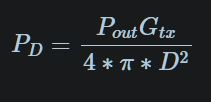GQ Electronics Technical Support Forum © Copyright since 2011Generated in 0.16 sec. Snitz's Forums 2000